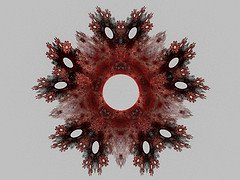## Tuesday, August 22, 2006

### Gravitons & SymmetryThe world can only
be grasped by action,
not by contemplation.
Jacob Bronowski

Without contemplation
how can you know
or enjoy what you
have grasped Quasar9
______________________________________________________
Picture Symmetry by cyberchaos @ flickr

Gravitons

In physics, the graviton is a hypothetical elementary particle that transmits the force of gravity in the framework of quantum field theory. If it exists, the graviton must be massless (because the gravitational force has unlimited range) and must have a spin of 2 (because gravity is a second-rank tensor field).

Gravitons are postulated because of the great success of the quantum field theory (in particular, the Standard Model) at modeling the behavior of all other forces of nature with similar particles: electromagnetism with the photon, the strong interaction with the gluons, and the weak interaction with the W and Z bosons. In this framework, the gravitational interaction is mediated by gravitons, instead of being described in terms of curved spacetime like in general relativity. In the classical limit, both approaches give identical results.

However, attempts to extend the Standard Model with gravitons run into serious theoretical difficulties at high energies (processes with energies close or above the Planck scale) because of infinities arising due to quantum effects (in technical terms, gravitation is nonrenormalizable.) Some proposed theories of quantum gravity (in particular, string theory) address this issue. In string theory, gravitons (as well as the other particles) are states of strings rather than point particles, and then the infinities do not appear, while the low-energy behavior can still be approximated by a quantum field theory of point particles. In that case, the description in terms of gravitons serves as a low-energy effective theory.
Since gravity is very weak, there is little hope of detecting single gravitons experimentally in the foreseeable future.

Gravitons & experiments

Detecting a graviton, if it exists, would prove rather problematic. Because the gravitational force is so incredibly weak, as of today, physicists are not even able to directly verify the existence of gravitational waves, as predicted by general relativity. (Many people are surprised to learn that gravity is the weakest force. The dominance of gravity at large scales is due to the fact that the nuclear forces have a limited range, and the electromagnetic force often largely cancels due to the existence of positive and negative charges. In contrast, gravitational charge -- i.e., mass -- is positive or zero for all known forms of matter.)

Gravitational waves may be viewed as coherent states of many gravitons, much like the electromagnetic waves are coherent states of photons. Projects that should find the gravitational waves, such as LIGO and VIRGO, are just getting started.

Is gravity like other forces?

Some question the analogy which motivates the introduction of the graviton. Unlike the other forces, gravitation plays a special role in general relativity in defining the spacetime in which events take place. Because it does not depend on a particular spacetime background, general relativity is said to be background independent. In contrast, the Standard Model is not background independent. In other words, general relativity and the standard model are incompatible. A theory of quantum gravity is needed in order to reconcile these differences.

Whether this theory should itself be background independent, or whether the background independence of general relativity arises as an emergent property is an open question. The answer to this question will determine whether or not gravity plays a "special role" in this underlying theory similar to its role in general relativity.

Gravitomagnetism

Sometimes called Gravitoelectromagnetism, (abbreviated GEM), refers to a set of formal analogies between Maxwell's field equations and an approximation to the Einstein field equations for general relativity, valid under certain conditions. For instance, the most common version of GEM is valid only far from isolated sources, and for slowly moving test particles.

This approximate reformulation of gravitation as described by general relativity makes a "fictitious force" appear in a frame of reference different from a moving, gravitating body. By analogy with electromagnetism, this fictitious force is called the gravetomagnetic force, since it arises in the same way that a moving electric charge creates a magnetic field, the analogous "fictitious force" in special relativity.

The main consequence of the gravetomagnetic force, or acceleration, is that a free-falling object near a massive rotating object will itself rotate.

This prediction, often loosely referred to as a gravitomagnetic effect, is among the last basic predictions of general relativity yet to be directly tested. A group at Stanford University is currently analyzing data from the first direct test of GEM, the Gravity B satellite experiment. Frame-dragging is often mentioned as a gravitomagnetic effect, but the Lense-Thirring effect (precession) may be a more appropriate example.
______________________________________________________

Plato: Gravitational Wave Detectors are Best
______________________________________________________